## Need help - Pink noise analyzer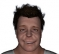Started by 4 years ago21 replieslatest reply 4 years ago131 views

Hello, I am making a 31 band pink noise analyzer.

I send the pink noise file through a windowed FFT. I then take the frequency data and add the values in each 1/3 octave together. If I understand Pink noise correctly I should have equal values in each octave (or a flat graph). But what I am getting is a slope with larger values towards the higher frequencies.

Here is an image of the 31 band's graphed.

Lowest value is:0.05139772 Highest value is 2.575877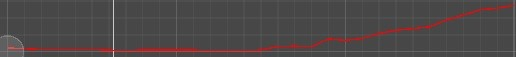Is there something I am missing or that I do not understand correctly?

Thanks

#### ANSWER: Below you will find the path to the current answer which is:

energy = (fftResults[i] - 1/f) ^ 2

OneThirdOctaveBin[Index] += energy;

Thanks to &

Also thanks to for the explanation on calculating Decibels.

dB = 20*log(magnitude)

Also thanks to everyone else who added to the discussion!

[ - ]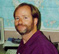You aren't calculating the energy correctly.

You want to square each FFT value (to convert from amplitude to energy), and then take the average of the points in each 1/3 octave.  I think you will find that this is flat for 1/f noise.

[ - ]That is getting me a flat response! Thank you...

I switched the code to this: (and divide the bin once full by the number of values I put in the bin.

float energy = Mathf.Pow(fftResultBins[i], 2);
bin[binIndex] += energy;

changed from:
bin[binIndex] += fftResultBins[i] - (1f / currentFrequency);

Pink noise graph: Black is un-smoothed, pink is smoothed.440hz graph: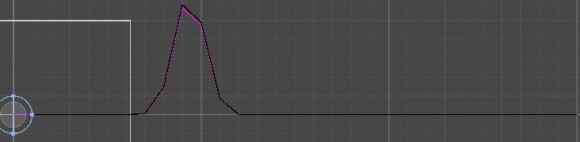White noise Graph:80hz, 440hz, 20k (looks like it is giving me the right results!)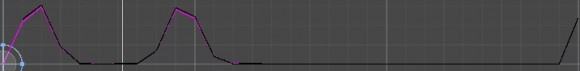Do the results look right? Did I understand correctly?

Thanks!

[ - ]I misspoke... Taking the mean of each 1/3 octave should make the white noise flat. Taking the sum of each 1/3 octave should make the 1/f noise flat. In other words, white noise has the same energy in each Hz, while 1/f noise has the same energy in each octave.

But in your graphs the 1/f noise and the white noise look the same... that can't be right.

[ - ]Here are the 2 noise types zoomed in with the mean. I see what you mean.

Pink is not flat but white is. I will try the other next.

white noisePink noise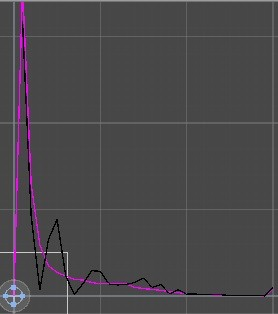[ - ]float energy = Mathf.Pow(fftResultBins[i], 2);
b[binIndex] += energy;

Without dividing by the number of values in the bin results in this.[ - ]Think I got it now.

float energy = Mathf.Pow(Mathf.Abs(fftResultBins[i] - (1f / currentFrequency)), 2);

b[binIndex] += energy;

PinkWhite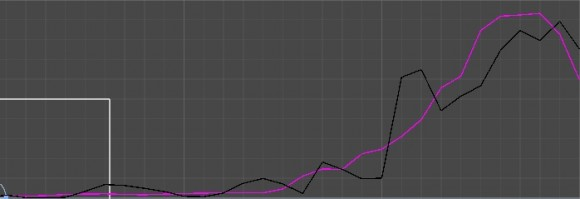[ - ]Pink Noise has a spectral density that decreases in 1/f.

so -10dB per octave

[ - ]Thanks for the quick reply! Did I understand correctly in the code below? I subtract 1/currentFrequency from each frequency that I am adding to the bins? I have no idea how to convert it to db to see if it is correctly subtracting 10db per octave.

void Make31OctaveBand()
{
float currentFrequency = 0;
int binIndex = 0;
bins[binIndex].value = 0;
hzPerBin = SamplingRate / (float)fftResultBins.Length;

for (int i = 0; i < fftResultBins.Length; i++)
{
currentFrequency = i * hzPerBin;
//if larger than bin's max frequency move to next bin
if (currentFrequency > bins[binIndex].maxFrequency)
{
binIndex++;
bins[binIndex].value = 0;
}
bins[binIndex].value += fftResultBins[i] - (1 / currentFrequency);
}
}

With this new code I get this. (red is the fftResults, magenta is my 31 band values)[ - ]If the red in your plot is the FFT results then that's not the FFT of typical pink noise.  BTW for any given FFT there is going to be significant variation (it's noise after all...) in each bin's level but in my experience averaging somewhere in the range of 8 to 128 frames will get you something smooth-ish.

[ - ]Does it not look right because it is linear? Here it is in log. Green is fft results and blue is 1/f. (The results are better but as seen above, the graph is still sloping up.) I'll try your suggestion with smoothing next. Thanks again!

Ps: can someone tell me how I convert the fftResults to DB?[ - ]It looks like your approach is wrong - you sum up FFT results for each 1/3 octave and expect the output to resemble 1/f scaling. However, the number of FFT bins per octave or 1/3 octave is not constant, so better divide each sum by the number of summands.
[ - ]Hi. Regarding your question: "how I convert the fftResults to DB?", the answer is: For each complex-valued FFT output sample you must compute that sample's magnitude value (recall Pythagoras' Theorem). Now you have a sequence of real-valued magnitude samples. Next, compute 20 times the log-base-ten of each of those magnitude sample values. That last step yields your spectral samples measured in dB.

[ - ]Thanks, I'll use some different code that allows me access to the imaginary and real parts. With the function I use now I only have access to the frequency bins.

[ - ]Hi FreeGameDev.

I went to the following web site:

I can't read the software gibberish on that page but I had the distinct impression that the 'GetSpectrumData' function generates spectral data that is in terms of dB.

FFT software produces output data in one of the following forms (formats):

* complex-valued data,

* rel-valued magnitude data,

* real-valued power data (magnitude squared),

* decibels (dB)

FreeGameDev, it's your job to determine which one of the above types of FFT output data is produced by the 'Get SpectrumData' function. Do need help in that determination?

[ - ]From this post here it says "each element shows the relative amplitude (0..1) of a the frequency equal to **N 24000 / Q* Hertz"

It looks like the values represented are amplitude. So, I guess the thing is to get DB from amplitude.

dB = 20*log( amplitude)  <- this right?

[ - ]Hi FreeGameDev

I believe what that post calls "amplitude" is what I call "magnitude." If that is true then you are correct, and you should use:

dB = 20*log(amplitude)

[ - ]Thanks

Now how do I give beers in here. :)

[ - ]Hi FreeGameDev.

Richard Lyons

Cell# 1638, Cell Block D

Folsom State Prison

Folsom, California  95603

[ - ]lol

[ - ]